# Bayesian Customer Lifetime Values Modeling using PyMC3

Modelingposted by ODSC Community June 21, 2023

Customer lifetime value (CLV) is the total worth of a customer to a company over the length of their relationship. The...Customer lifetime value (CLV) is the total worth of a customer to a company over the length of their relationship. The collective CLV of a company’s customer base reflects its economic value and is often measured to evaluate its future prospects.

While many ways to estimate CLV exist, one of the most influential models to surface in the past couple of decades is the Beta-Geometric Negative Binomial Distribution (BG-NBD).¹ This framework models customers’ repeat purchasing behavior using the Gamma and Beta distributions. Part 1 of this series of articles already explored the mathematics behind this model. Meanwhile, part 2 introduced lifetimes, a library that allows us to conveniently fit a BG-NBD model and obtain its maximum likelihood estimate (MLEs) parameters. I’d suggest you read these parts if you haven’t as the upcoming content builds on top of them.

This last part of the series presents an alternative way to implement BG-NBD — one that relies on Bayesian principles. We’ll be using PyMC3 to code this implementation. While the principal aim of this article is to elucidate BG-NBD through the Bayesian lens, some general points on the Bayesian worldview and PyMC3 framework will also be presented. Here is our agenda:

1. First, we’ll argue that the Bayesian implementation offers some advantages over the frequentist.
2. Second, we’ll provide an implementation ‘blueprint’ that will guide our coding steps.
3. Third, we’ll spend some time looking into prior selection — a key component in Bayesian modeling.
4. Next, we’ll go over the PyMC3 code and its intricacies, including the famous Monte-Carlo Markov Chain (MCMC) algorithm.
5. Finally, we’ll analyze the output of our Bayesian BG-NBD model and compare it with the output of lifetimes.

Let’s get started!

## Why Bayesian?

You might be wondering as to why there is a need for another implementation if we already have the user-friendly lifetimes in our arsenal. The answer lies in the contrast between frequentist and Bayesian inference and the advantages offered by the latter.

To illustrate these differences, let’s imagine the frequentist and Bayesian frameworks as two programming functions that attempt to model statistical problems (such as CLV estimation). We’ll see that these functions have crucial differences in their inputs and outputs.

Both functions take as an input the dataset (the observations). However, the Bayesian function requires additional inputs in the form of prior distributions. Priors reflect our initial assumptions of the parameters of the models and will be updated after the observations have been taken into account.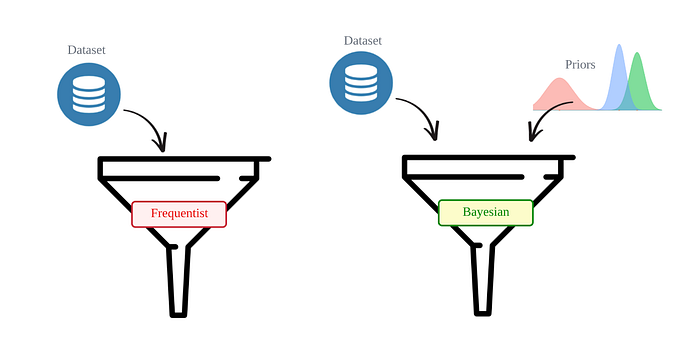The inclusion of priors is a double-edged sword. On the one hand, priors offer a way for users to use their domain knowledge to ‘guide’ the model into finding the correct parameters. In BG-NBD for example, if we’re sure that most of our customers make purchases at a rate λ of 4 transactions/week, we can supply priors that would lead to a Gamma distribution with a mean of 4. If this intuition is only slightly off-the-mark (for example, if the real λ is 3.5 transactions/week), the Bayesian framework will allow us to arrive at the correct values with only few data points.

On the other hand, since there isn’t a single ‘correct’ way to choose the priors, the Bayesian modeling process is subjective and variable. Two statisticians sharing the same data but employing different priors could end up getting completely different outcomes. This subjectivity is among the chief reasons why some practitioners eschew Bayesian. Nevertheless, as more data points are included in the modeling, the influence of priors diminishes.

The two functions also differ in their outputs. They both return the parameter estimates of the model but in different forms. The frequentist returns them as point estimates. For example, we’ve seen how the frequentist library lifetimes use MLE to calculate the point estimates of r, α, a, and b. In contrast, the Bayesian returns them as probability distributions. These distributions, called posteriors, represent our updated beliefs about the parameters after taking into account the observations.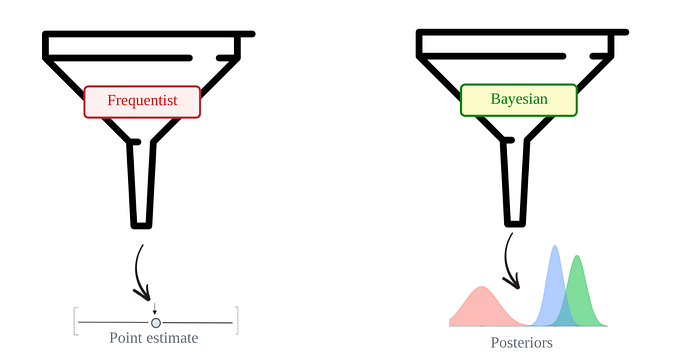The obtainment of posteriors is another aspect where the Bayesian framework outshines the frequentist. With posteriors, it is easy to visualize the most plausible values for the parameters and quantify any uncertainty in our estimates. Additionally, the prior-to-posterior cycle provides a principled way to update the parameters if additional observations become available.

## Implementation Blueprint

We’ve seen in Part 1 that the BG-NBD model is a hierarchical probabilistic model. Hierarchical here refers to the fact that the parameters of some distributions are modeled by other distributions (‘parent’ distributions). We’ll soon see that PyMC3 allows us to express these parent-child relationships in code.

A blueprint is provided below to guide the coding steps. This blueprint walks through the model’s logical flow, starting from its inputs (the data and the prior distributions) and ending with its outputs (the posteriors).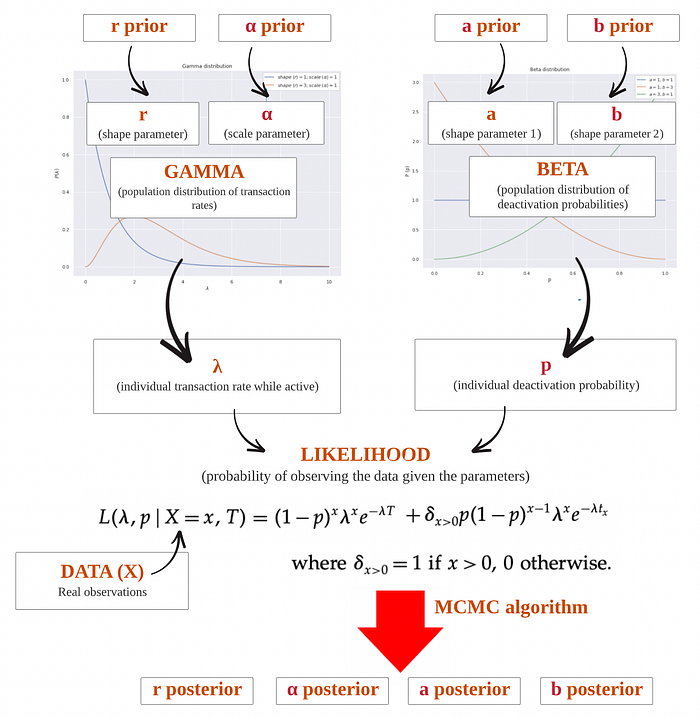Some remarks on the above blueprint:

1. The Gamma distribution represents the distribution of transaction rate λ in the customer population. It is parameterized by two parameters: r and α. We’ll supply priors for both r and α that will be updated by our observations.
2. The Beta distribution represents the distribution of deactivation probability p in the customer population. It is parameterized by two shape parameters: a and b. Similarly, we’ll provide priors for these parameters.
3. The λ from the Gamma distribution and the p from the Beta distribution contribute to the likelihood function, which represents the probability of observing the transactions in the dataset. This likelihood is the equation that binds our data and our theory.
4. An MCMC algorithm in PyMC3 will enable us to sample the posterior distributions of r, α, a, and b. We’ll talk more about this algorithm later.

Details about the above distributions were provided in part 1; take a look at it if you’re unfamiliar with them!

## Selection of priors

### Consideration

As discussed previously, we’ll need to supply a prior each for r, α, a and bWe can think about these priors and their respective parameters as the ‘hyperparameters’ of our model. There are various considerations to keep in mind when choosing a prior:

1. A prior can be formulated from existing knowledge, which in turn can come from domain expertise, historical observations or past modeling experiments.
2. When no information is available, an uninformative prior can be chosen to reflect an indifference among possible parameter values.
3. We can impose some constraints to guide our selection. For example, we can require that our prior distributions be symmetrical, strictly positive, heavy tailed, etc.

In our case, the following are our considerations and assumptions:

1. As for the purchase rate λ, we’ll assume that we have a strong intuition that the bulk of our customer transact at a rate of 4 purchases/week. We’ll select Gamma priors that reflect this prior belief.
2. In contrast, we’ll assume no prior knowledge on the deactivation probability (p) of our customers. As such, we’ll choose uninformative Beta priors.
3. Because all of our parameters (r, α, a and b) can only be positive real numbers, the prior distributions we choose must assign zero probability density for negative numbers.

### Truncated Normal Distribution

A good candidate for the priors is the Truncated Normal distribution. As its name suggests, the Truncated Normal is a Gaussian distribution that has been ‘truncated’. That is, its density beyond a specified left and a right limit is set to zero and its density between the limits is re-normalized to sum to 1. The distribution has four parameters: the left and right limits (often referred to as and B), the mean μ and the standard deviation σ.

Truncated Normal is frequently used as prior as its four parameters provide an intuitive way to ‘guess’ a value. μ represents our most probable guess, σ reflects our uncertainty level and and B designate the lower and the upper constraint, respectively.

Here are some examples of the Truncated Normal: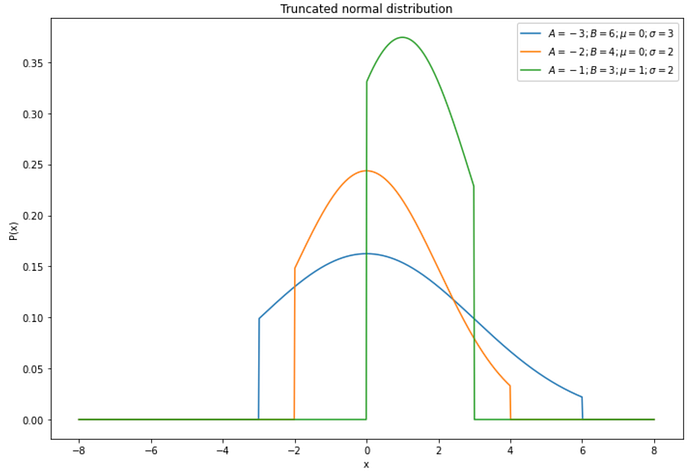### Gamma and Beta Priors

With these considerations, let’s now construct our Gamma priors. Here are the steps:

1. As mentioned before, we have a prior belief that the most likely value of the Gamma distribution (i.e. its mean) is 4 transactions/week.

2. We then select a specific combination of the Gamma parameters and α that leads to a Gamma distribution with the desired mean of 4. We know that a Gamma distribution’s mean can be calculated by the simple formula μ = rα; as such, any pair of positive numbers whose product is 4 can be chosen as candidates for and α. Let’s set to be 8 and α to be 0.5. The Gamma distribution built using these parameters is plotted below: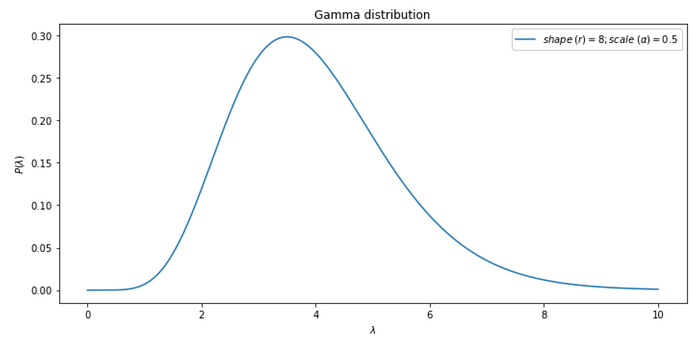3. These and α values represent our initial guesses. Since we’re not 100% sure of these guesses, we should present them as distributions as opposed to point estimates. It is these distributions that we refer to as priors. For reasons previously mentioned, we’re going to use the Truncated Normal to represent these priors.

4. Now let’s focus on r. The Truncated Normal for r will have the following parameters:

• μ: 8 (our most probable initial guess)
• σ: 7 (a rather wide standard deviation that reflects our high uncertainty level)
• A: 0 (since we know that can’t be negative)
• B: 40 (here, we pick an extreme right limit which we are won’t exceed).

The Truncated Normal built using these parameters is plotted below: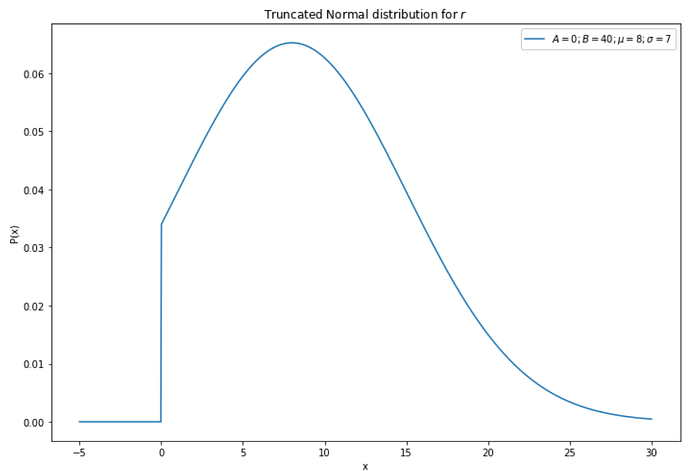5. The above process is repeated to get the priors for α, a and b. The workflow is visualized below: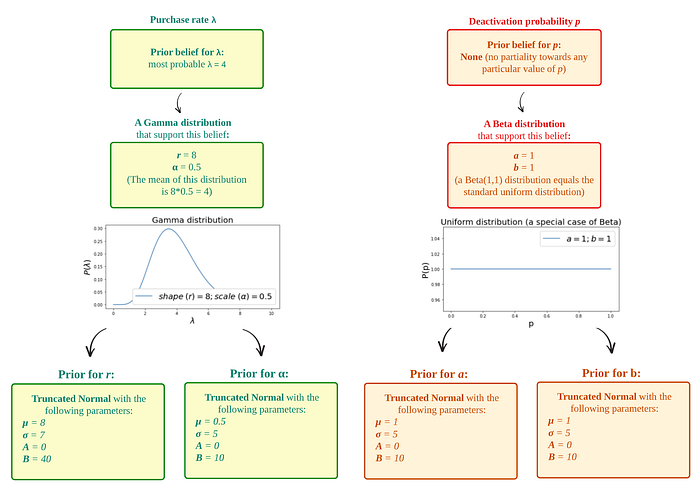Note that for the Beta parameters and b, we have chosen the uninformative uniform distribution as priors to reflect that we have no prior knowledge on the deactivation probability (p) of our customers.

## Coding everything in PyMC3

### Dataset

Throughout this article, we will be using the sample dataset (MIT licensed) provided by lifetimes. This table records the transactions of CDNow, a dot-com era company that sold CDs. Here is how we load it:

```import pandas as pd

filename='CDNOW_sample.txt',
delim_whitespace=True,
names=['customer_id', 'customer_index', 'date', 'quantity', 'amount'],
converters={'date': lambda x: pd.to_datetime(x, format="%Y%m%d")}
)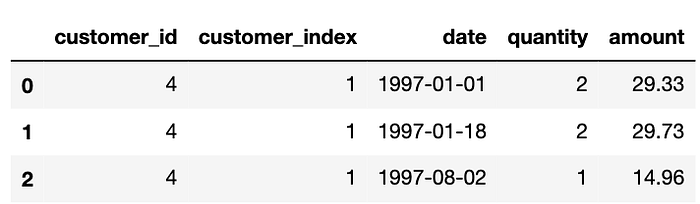transactions

As discussed in part 2, prior to modeling, this transactions table should be subjected to two prerequisite steps:

1. converted to the canonical ‘RFM’ format, and
2. split into the calibration and holdout sets.

We’ll employ the same splitting regime we used in part 2 to facilitate the comparison between the lifetimes and the PyMC3 estimates.

```from lifetimes.utils import calibration_and_holdout_data

rfm_cal_holdout = calibration_and_holdout_data(transactions=transactions,
customer_id_col='customer_id',
datetime_col='date',
monetary_value_col = 'amount',
freq='W',
calibration_period_end='1998-01-01',
observation_period_end='1998-06-30' )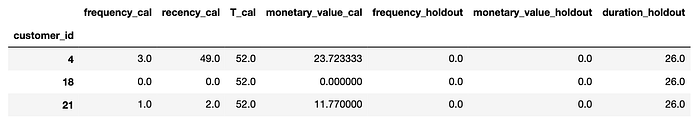rfm_cal_holdout

### PyMC3 in action

With this theoretical framework set up, we’re now ready to start coding. We’ll use PyMC3, a powerful Bayesian modeling library. While we’ll provide a few pointers on PyMC3 itself, our focus will be on the BG-NBD implementation².

In PyMC3, a probabilistic model is represented by a pm.Model() object which is usually coded as a context manager. This model will contain several distributions represented by Distribution objects. These Distribution objects can have parent-child relationships among themselves, where the value of the parent object influences/determines the value of the child object.

The following is the BG-NBD implementation in PyMC3. As you can see, the code closely mirrors the theory presented earlier.

```from pymc3.math import log, exp, where
import pymc3 as pm
import numpy as np

# We use the "calibration" portion of the dataset to train the model
N = rfm_cal_holdout.shape # number of customers
x = rfm_cal_holdout['frequency_cal'].values # repeat purchase frequency
t_x = rfm_cal_holdout['recency_cal'].values # recency
T = rfm_cal_holdout['T_cal'].values # time since first purchase (T)

# Modeling step
bgnbd_model = pm.Model()
with bgnbd_model:

# Priors for r and alpha, the two Gamma parameters
r = pm.TruncatedNormal('r', mu=8, sigma=7, lower=0, upper=40)
alpha = pm.TruncatedNormal('alpha', mu=0.5, sigma=5, lower=0, upper=10)

# Priors for a and b, the two Beta parameters
a = pm.TruncatedNormal('a', mu=1, sigma=5, lower=0, upper=10)
b = pm.TruncatedNormal('b', mu=1, sigma=5, lower=0, upper=10)

# lambda_ (purchase rate) is modeled by Gamma, which is a child distribution of r and alpha
lambda_ = pm.Gamma('lambda', alpha=r, beta=alpha, shape=N, testval=np.random.rand(N))

# p (dropout probability) is modeled by Beta, which is a child distribution of a and b
p = pm.Beta('p', alpha=a, beta=b, shape=N, testval=np.random.rand(N))

def logp(x, t_x, T):
"""
Loglikelihood function
"""
delta_x = where(x>0, 1, 0)
A1 = x*log(1-p) + x*log(lambda_) - lambda_*T
A2 = (log(p) + (x-1)*log(1-p) + x*log(lambda_) - lambda_*t_x)
A3 = log(exp(A1) + delta_x * exp(A2))
return A3

# Custom distribution for BG-NBD likelihood function
loglikelihood = pm.DensityDist("loglikelihood", logp, observed={'x': x, 't_x': t_x, 'T': T})

# Sampling step
SEED = 8
SAMPLE_KWARGS = {
'chains': 1,
'draws': 4000,
'tune': 1000,
'target_accept': 0.7,
'random_seed': [
SEED,
]
}
with bgnbd_model:
trace = pm.sample(**SAMPLE_KWARGS)

# It's a good practice to burn (discard) early samples
# these are likely to be obtained before convergence
# they aren't representative of our posteriors.
trace_trunc = trace[3000:]```

Having said that, two parts of the code might not be as straightforward and warrant further discussion. These parts, the custom likelihood function (line 30–41) and the MCMC sampling (line 43–62), are elaborated below.

### Custom likelihood function

PyMC3 is equipped with many Distribution objects, each of which corresponds to a well known probability distribution such as the Beta or Gamma distribution. However, when it comes to a non-standard probability distribution such as the BG-NBD likelihood function, custom implementation is required.

As a reminder, the likelihood function in the BG-NBD model is shown below (its derivation was provided in part 1):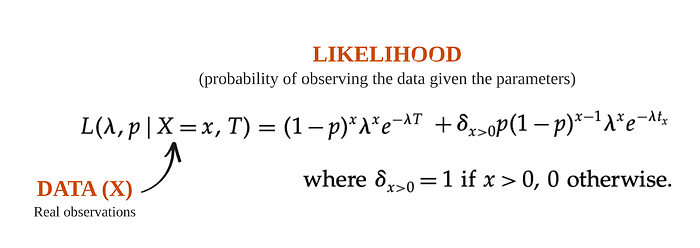You might remember from your statistics class that the maximum likelihood estimation (MLE) involves the multiplication, over our entire dataset, of the outputs of the likelihood function. Since each of these multiplication steps outputs a fraction, a direct calculation of likelihood might lead to floating point problems. To avoid this, it is customary to convert the likelihood function into a log-likelihood function, which is more convenient to optimize. The log-likelihood version of the above function is as follows: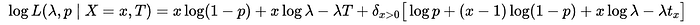It was this above formula that we implemented in the logp function. We then turned this function into a Distribution object by instantiating a new DensityDist object and passing on the callable during the instantiation. Because this Distribution object is one whose values are known, we also supplied these known values the tₓ and T from our data) during its instantiation.

## The MCMC algorithm

After describing the model, we can execute an MCMC algorithm to sample the posterior distributions. MCMC is a family of simulation algorithms that allows the sampling from a distribution whose closed-form PDF or PMF is unknown. It works by constructing a Markov chain — a multi-state system that allows transitions from one state to another according to specific probabilistic rules. The chain is designed so that its steady state matches the distribution to be sampled.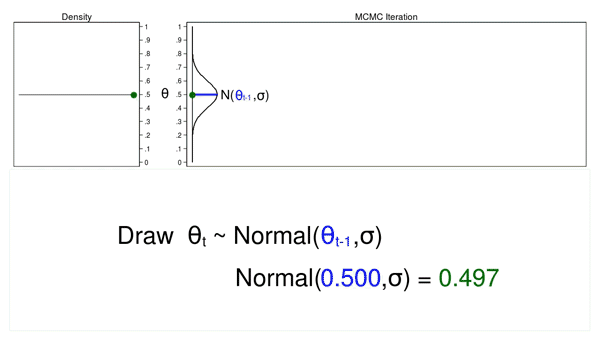Animation of MCMC from the Stata Blog³

We start the chain construction and sampling using pm.sample(). Upon completion, the function returns a trace object that contains the drawn samples. If the sampling process went well (i.e. a steady state was reached), these samples would represent our desired posteriors.

Before further analysis, we should confirm the convergence (the steady-state attainment) of the algorithm, as using samples obtained before convergence could lead to erroneous downstream analyses. To ascertain convergence, we can plot trace using pm.trace_plot()⁴; a ‘meandering’ pattern indicates non-convergence and is no bueno.

Here is the trace plot for our experiment: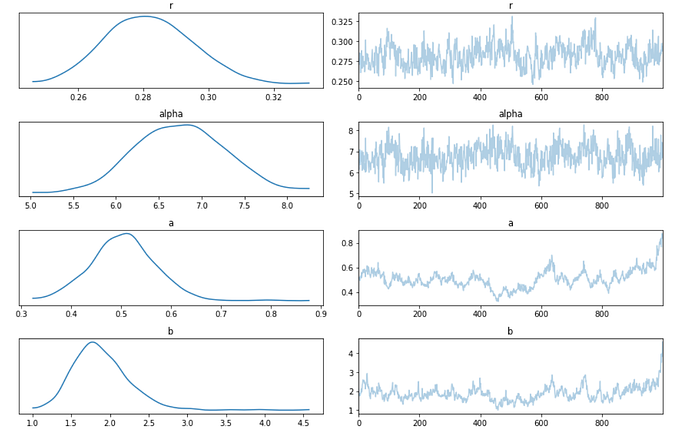We see that no obvious sign of non-convergence was detected.

## Posterior distribution analysis

### Priors vs posteriors

Since we’re confident that the sampling process ran properly, we can proceed to analyze the posteriors. A common starting point is pm.summary(), which returns summary statistics of our posteriors. Let’s print their means.

```params_mean = pm.summary(trace_trunc, var_names = ["r", "alpha", "a", "b"])["mean"]
params_mean```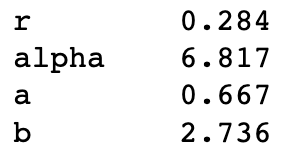We note that these posterior means are quite different from the prior means. For instance, goes from 1 (prior mean) to 2.736 (posterior mean). This indicates that our prior beliefs were not fully supported by the data and thus were ‘updated’.

In the previous article of the series, we’ve seen the use of lifetimes library can also be used to estimate the parameters r, αa and b. Let’s now compare the result from the two implementations: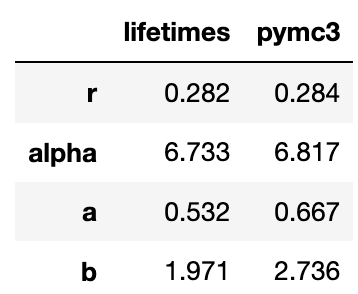We see that despite coming from different frameworks (frequentist vs Bayesian), the estimated parameters are not that different.

## Analysis of estimated Beta and Gamma distributions

We can then use the mean (i.e. the most probable) parameter values of the Bayesian posteriors to construct and visualize the Gamma and Beta distributions.

Let’s start from Gamma — here is the graph: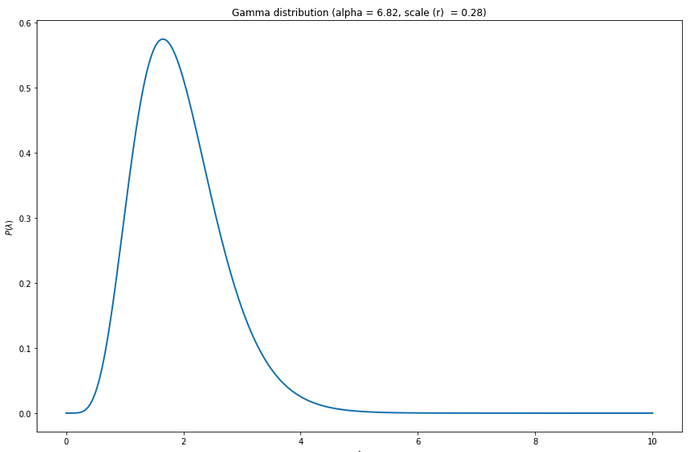As we discussed in part 1, the Gamma distribution has a practical significance — it quantitatively describes the collective purchasing behavior of our customer base. Specifically, it indicates the distribution of purchasing rates within the population. The plot above displays a relatively desirable Gamma distribution with most of the 𝜆 found near 2. This means our customers are expected to shop at a rate of 2 transactions per week — not a bad rate for a CD company!

Now let’s look at the Beta distribution, which describes the distribution of the deactivation probabilities within our customer base: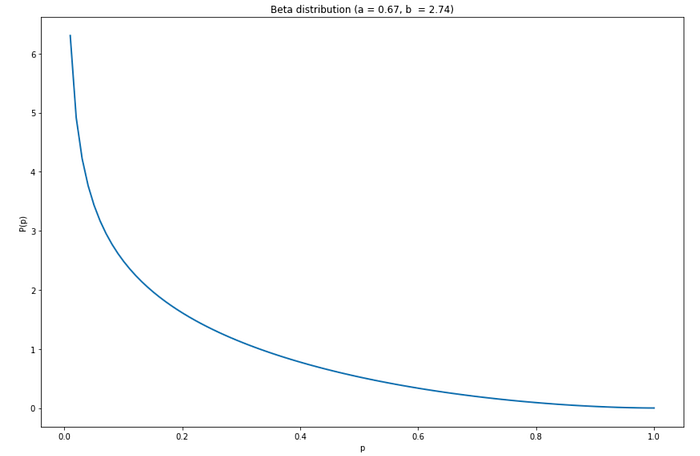The plot displays a relatively healthy Beta distribution that concentrates most of its near 0. This implies that as a whole, our customers are unlikely to deactivate.

## Conclusion

In this series of articles, we’ve discussed the fundamentals BG-NBD model and showcased its value in tackling real-world business problems.

Specifically, in article 1, we learned that BG-NBD models the repeat purchase behavior and the deactivation probability of the customer population. The resulting probability distributions are not only theoretically sound; they also present insightful quantitative representations of our individual customers and customer base.

In article 2, we explored lifetimes, the frequentist implementation of BG-NBD. lifetimes allows us to fit a BG-NBD model with several lines of code. We’ve also seen a couple of practical ways to use the outputs from lifetimes. I’d recommend lifetimes for analysts who don’t wish to manually code the BG-NBD equations and want to start deriving business values immediately.

This third and last article implemented BG-NBD using the Bayesian PyMC3 library. This implementation involves coding BG-NBD from scratch and as such requires a more in-depth understanding of the model. The advantage is that it allows us to specify the priors, and this allows us to incorporate our expert knowledge into the modeling process. I’d recommend this approach for practitioners having both deep familiarity with BG-NBD and domain knowledge of the customer base they are trying to model.

Article originally posted here by Meraldo Antonio. Reposted with permission.## ODSC Community

The Open Data Science community is passionate and diverse, and we always welcome contributions from data science professionals! All of the articles under this profile are from our community, with individual authors mentioned in the text itself.

1